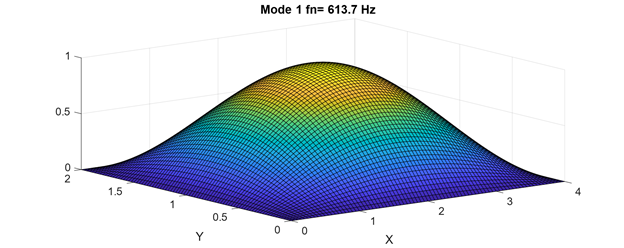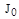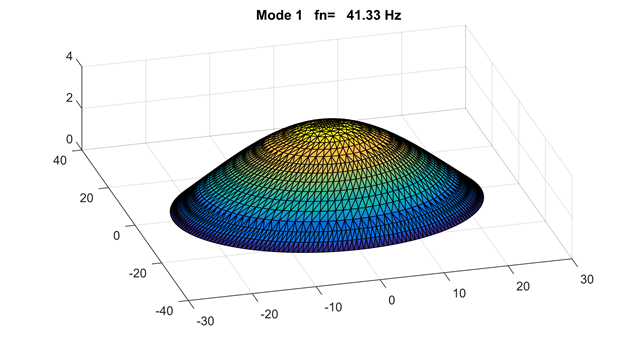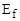Thin Plate Vibration
By Tom Irvine
SECTION 1
Rectangular Plate, Bending Vibration
Rectangular Plate Equations

The fourth-order governing equation of motion for the out-of-plane displacement is

The plate stiffness factor is

The kinetic energy in the plate is

The potential or strain energy in the plate is

Assume a harmonic displacement response for the partial differential equation (1.1).

Substitution of the assumed response leads to the spatial equation

The modal solution equation (1.6) for most boundary conditions requires an approximation method such as the Rayleigh-Ritz or finite element method. Further information on plate vibration solutions is given in Reference .

Rectangular Plate, Simply-Supported along All Edges

The case of plate with simply-supported boundary conditions on all four edges can be solved for exactly. The boundary conditions for this plate are

The displacement and bending moment along each edge is equal to zero.

Assume the following displacement function which satisfies the boundary conditions, where and are indices. The mass-normalized modes shapes for the plate are

The angular natural frequencies are

Rectangular Plate Example

Consider a rectangular circuit board made from G10 material. The modulus of elasticity is 2.7e+06 lbf/in^2 with Poisson ratio of 0.12. The dimensions of the circuit board are 4 in x 2 in x 0.063 in. The board is simply-supported about its perimeter. Assume that the electronic components do not add any stiffness. The board has a uniform mass distribution. The total mass is 0.113 lbm. This includes the G10 board and the electronic components. The mass per area is

The circuit board stiffness factor is

The fundamental angular natural frequency is

The fundamental frequency is equivalent to 613.7 Hz. The mode shape is shown in the following figure.Figure 1.2. Circuit Board, Fundamental Bending Mode Shape
SECTION 2
Circular Plate, Bending Vibration
Circular Plate Equations

The governing fourth-order spatial equation of motion for the out-of-plane displacement is

The biharmonic operator is

The wave number is

The kinetic energy in the plate is

The potential or strain energy in the plate is

The solution of equation (2.1) proceeds by assuming separation of the spatial variables.

Further information on this solution method is given in Reference .

Circular Plate, Simply-Supported

The boundary conditions for the simply-supported plate are

The mass-normalized, fundamental mode shape is

Note thatandin equation (2.9) are Bessel functions. This fundamental mode shape is sometimes referred to as an “oil can” mode. The corresponding fundamental frequency is

A characteristic equation for variable mode numbers and Possion ratios is given in Reference . The roots of this equation determine the numerical coefficient on the righthand side of equation (2.10).

Circular Plate Example, Simply-Supported

Assume a 48 inch diameter, 0.5 inch thick, aluminum circular plate, with a simply-supported circumference. Calculate the fundamental frequency and mode shape.

The plate stiffness factor is

The mass per area is

The angular natural frequency is

The angular frequency is equivalent to 41.33 Hz.Figure 2.2. Circular Plate Fundamental Mode Shape
SECTION 3
Honeycomb Sandwich Plate
Honeycomb Sandwich Plate Characteristics

Honeycomb sandwich structures are designed to have a high stiffness-to-mass ratio. The stiff, strong face sheets carry the bending loads, while the core resists shear loads. The face sheets are typically made from aluminum or carbon fiber with epoxy resin. The honeycomb core material is usually aluminum for aerospace applications. Other core materials include Nomex aramid or Kevlar para-aramid fiber sheets saturated with a phenolic resin. In addition, closed cell foams such as Rohacell are substituted for honeycomb in some sandwich panel designs.

Table 3.1. Honeycomb Sandwich Plate Normal Modes
Domain Characteristic
Low Frequencies Bending of the entire structure as if were a thick plate
Mid Frequencies Transverse shear strain in the honeycomb core governs the behavior
High Frequencies The structural skins act in bending as if disconnected

The modal response of honeycomb sandwich plates has the characteristic shown in Table 3.1. The lower natural frequencies may be calculated using the plate formulas in Sections 9.1.1 and 9.1.2 by properly accounting for the material and geometric properties.

Assume

• The skin elastic modulus is much greater than the core modulus.
• Each skin has the same material.
• Each skin is "thin" relative to the core.

The honeycomb sandwich plate bending stiffness from Reference  is

The elastic modulus of each skin or face sheet is.

Honeycomb Sandwich Plate Example, Part I

A suborbital rocket vehicle has a circular bulkhead made from honeycomb. The material is aluminum for both core and skins. The bulkhead properties are shown in the following table. Calculate the fundamental frequency of the bare bulkhead.

Table 3.2. Bulkhead Parameters
Parameter Value
Boundary Condition Simply Supported
Diameter 40 inch
Core Thickness 1.0 inch
Thickness of Each Skin 0.063 inch
Total Thickness 1.125 inch
Skin Elasticity 10.0e+06 lbf/in^2
Core Elasticity Negligible
Skin Density 0.10 lbm/in^3
Core Density 0.01 lbm/in^3
Poisson's Ratio 0.3

The plate stiffness is calculated in two steps.

The total skin mass is

The core mass is

The total bulkhead mass is

The total mass per area is

The angular fundamental frequency of the bare bulkhead for μ=0.33 is

The angular frequency is equivalent to 164 Hz. The mode shape is qualitatively similar to that in Figure 2.2.

Honeycomb Sandwich Plate Example, Part II

Now assume that 100 lbm of avionics components are added to the bulkhead and that this nonstructural mass is spread uniformly across the bulkhead. Neglect the geometry and stiffness of the avionics. Repeat the natural frequency calculation.

The total mass per area is